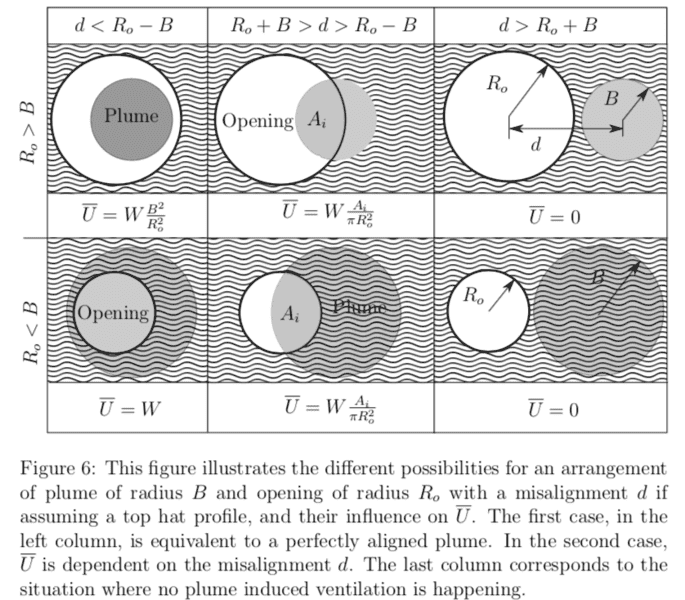# How to write this in Set Theory notation?

• danielFiuza
In summary, the intersection of two sets cannot be larger than either set. It can be represented by the notation A ∩ B, and in this case, A ∩ B = B ∩ A. If one set is completely contained within the other, the intersection will be the smaller set. This can be expressed as A ∩ B = A or A ∩ B = B, depending on which set is smaller.

#### danielFiuza

Hello Everyone,

I am trying to write the intersection of a physical problem in the most compact way.
I am not really familiar with Set Theory notation, but I think it has the answer.

It is about the intersection of two circular areas:
- Area 1: A
- Area 2: B
If I want to write this in Set Theory notation:
A_intersection = A ∩ B

Until this point everything is okay. But I have an extra condition
- Extra condition: intersection area always smaller than B (or in other words, the intersection area needs to be contained in B).
A_intersection = min{A ∩ B, B}
(ie. The maximum intersection area can not be larger than B)

How can I write this? Is there any short mathematical symbol for that?

Kind regards,

Daniel

PS: Physically, I am trying to describe a situation as the following one:
' A jet of water of radius R1 impinging against a wall with a hole of radius R2, misaligned from the opening. The water is driven through the intersection area, given that the intersectio area is smaller always that the hole radius R2'
1) For a perfectly aligned jet with the opening:
- If the radius of the jet R1 is smaller than the opening radius R2, all the fluid from the jet goes inside. -> R1
- If the area of the jet R1 is larger than the hole area R2, only the area R2 drives fluids-> R2
2) Then extending this for different misalignments.#### Attachments

Last edited:
danielFiuza said:
Hello Everyone,

I am trying to write the intersection of a physical problem in the most compact way.
I am not really familiar with Set Theory notation, but I think it has the answer.

It is about the intersection of two circular areas:
- Area 1: A
- Area 2: B
If I want to write this in Set Theory notation:
A_intersection = A ∩ B

Until this point everything is okay. But I have an extra condition
- Extra condition: intersection area always smaller than B (or in other words, the intersection area needs to be contained in B).
A_intersection = min{A ∩ B, B}
(ie. The maximum intersection area can not be larger than B)

How can I write this? Is there any short mathematical symbol for that?

The intersection of two sets cannot be larger than either set. We have:

##A \cap B \subseteq A## and ##A \cap B \subseteq B##

This follows because the intersection is only things that are in each set. By definition, therefore, the intersection of two sets is a subset of each set.

•danielFiuza
danielFiuza said:
But I have an extra condition
- Extra condition: intersection area always smaller than B (or in other words, the intersection area needs to be contained in B).
A_intersection = min{A ∩ B, B}

As to containment, it is always true that ##A \cap B \subseteq B##, so you don't need to state this as an additional condition.

If you want to require that ##A \cap B ## be a proper subset of ##B##, it's safest to write this as ##A \subsetneqq B##. (Some people interpret ""##\subset##"" to mean the same things as "##\subseteq##", others interpret it to mean "##\subsetneqq##" ).

Thanks Stephen. Just to be clear, is ##A \cap B = B \cap A ##?PeroK said:
The intersection of two sets cannot be larger than either set. We have:

##A \cap B \subseteq A## and ##A \cap B \subseteq B##

This follows because the intersection is only things that are in each set. By definition, therefore, the intersection of two sets is a subset of each set.

#### Attachments

danielFiuza said:
Thanks Stephen. Just to be clear, is ##A \cap B = B \cap A ##?

If you imagine drawing your sets on a Venn diagram, where ##A \cap B## is the area of overlap between ##A## and ##B##; and, ##B \cap A## is the area of overlap between ##B## and ##A##; then, would you say these represent the same area?

Last edited:
danielFiuza said:
Thanks Stephen. Just to be clear, is ##A \cap B = B \cap A ##?
View attachment 232588

Is the set of males over 20 years of age the same as the set of people over 20 years of age who are male?

PeroK said:
If you imagine drawing your sets on a Venn diagram, where ##A \cap B## is the area of overlap between ##A## and ##B##; and, ##B \cap A## is the area of overlap between ##B## and ##A##; then, would you say these represent the same area?

Yes, they represent the same area.
I am still confused when the one of the areas is completely contained inside the other (see first column of the diagram attached in the thread). Eg. A belongs to/is contained in B or area B belong to A. Does that still holds? That's were my confusion arises with the notation of ##\cap##.

Thanks again,

danielFiuza said:
Yes, they represent the same area.
I am still confused when the one of the areas is completely contained inside the other (see first column of the diagram attached in the thread). Eg. A belongs to/is contained in B or area B belong to A. Does that still holds? That's were my confusion arises with the notation of ##\cap##.

Thanks again,

In the case where ##A \subseteq B##, then the intersection of the two sets is the set ##A##.